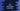# C program to print all printable characters## C program to print all printable characters:

A character is called printable character if it occupies a printing position. These characters includes all characters with ASCII value greater than 0x1f excluding 0x7f.

In C, we can check if a character is printable or not. There is a method called isprint, defined in ctype header, which we can use to verify if a character is printable.

In this post, we will learn how to print all printable characters in C.

## isprint method:

isprint method is defined as like below:

``int isprint(int c)``

It takes one integer value as the argument. This is the ASCII value of a character that we need to pass. If we pass a character, it will convert that character to integer internally.

It returns one integer value. It returns one non-zero value, if it is a printable value. Else, it returns 0.

### How to use isprint():

To use isprint, we need to import ctype.h header file. We can use it in a program after that.

Let me show you with an example:

``````#include <stdio.h>
#include <ctype.h>

int main()
{
printf("Is printable 'c' : %d\n", isprint('c'));
printf("Is printable '\t' : %d\n", isprint('\t'));

return 0;
}``````

If you run this program, it will print the below output:

``````Is printable 'c' : 1
Is printable '  ' : 0``````

You can see that for ‘c’, it is printing ‘1’ and for tab, it is printing 0.

We can also print all printable characters using isprint method. Using one loop, we can iterate through the ASCII numbers and if isprint returns non-zero value, we can print that character.

``````#include <stdio.h>
#include <ctype.h>

int main()
{
int ch;

for (ch = 1; ch <= 127; ch++)
{
if (isprint(ch) != 0)
printf("%c ", ch);
}

return 0;
}``````

This program is printing all printable characters. If you run it, it will give the below output:

``  ! " # \$ % & ' ( ) * + , - . / 0 1 2 3 4 5 6 7 8 9 : ; < = > ? @ A B C D E F G H I J K L M N O P Q R S T U V W X Y Z [ \ ] ^ _ ` a b c d e f g h i j k l m n o p q r s t u v w x y z { | } ~``